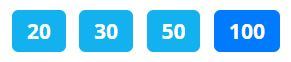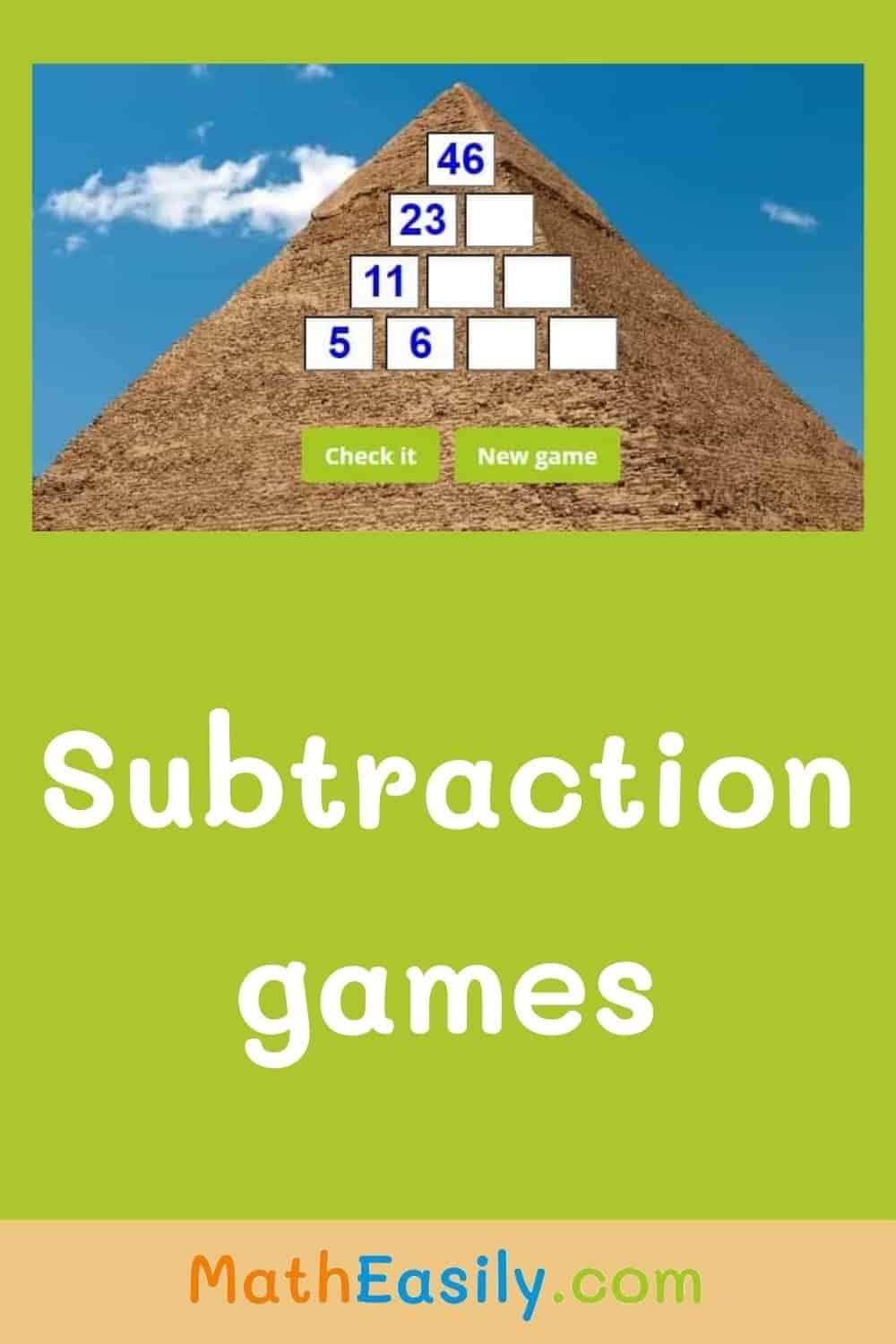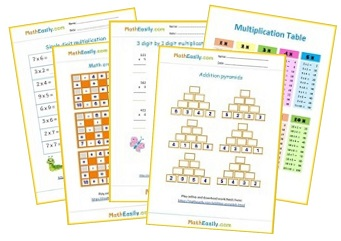﻿ 100+ Free Online Subtraction Games for Kids

Home > Subtraction Games

# Free Online Subtraction Games for Kids

Enjoy our online subtraction games for kids. 100+ Math subtraction exercises for Kindergarten and Grade 1-5. Check results, find mistakes. Play interactive subtraction games online.

Students need a lot of practice to master math. Therefore all these math subtraction games are accompanied by at least 3 worksheets on which kids can test their subtraction skills. Click the pictures below, play free subtraction games or download worksheets.

## Online Subtraction Games for Kindergarten

Play here

### Subtraction to 20 with pictures

Play here

Online Kindergarten Games →

## Interactive Subtraction Games for Grade 1

### Subtraction pyramids to 100

Play subtraction games online. Subtraction pyramids are great for learning subtraction.
Play here

### Math crossword puzzles

Play here

All Games for 1st Grade →

## Free Subtraction Games for Grade 2

### Two digit subtraction

Practice subtraction within 100 with our colored worksheets, play online games, find mistakes, play again.

Play here

### Math puzzles

Play here

All Games for 2nd Grade →

Our most popular free subtraction games for 3rd Grade are subtraction pyramid puzzles or these big math puzzles. You can choose the maximum value of these puzzles: 20, 30, 50 or 100. Third grade math students will prefer the highest value of 100:Other online subtraction games for Grade 3 will be added soon. In the meantime you can check out these online math games for 3rd Grade →## Math Subtraction Games Online

Our online subtraction games for kids teach subtraction. You can find here free subtraction games for Kindergarten and Grade 1-5.

Kids will immediately see the correct answer, they can learn from their mistakes.

Our interactive subtraction games are full of nice pictures, kids will have fun. Pin it for later!Free Math Worksheets